## Filters

Sort by :
Q
Engineering
470 Views   |

Which of the following factors is of no significance for roasting sulphide ores to the oxides and not subjecting the sulphide ores to carbon reduction directly?

• Option 1)

is more volatile than

• Option 2)

Metal sulphides are thermodynamically more stable than

• Option 3)

is thermodynamically more stable than

• Option 4)

Metal sulphides are less stable than the corresponding oxides

As we learnt in Roasting - It is heating of the ore in presence of air below its fusion temperature. - wherein 2PbS +3O3  2PbO + 2SO2   The reduction of metal sulphides by carbon reduction has positive   whereas,  is negative for the same process with metal oxides. So, metal sulphides are more stable than oxides.    Option 1) is more volatile than  This option is incorrect. Option 2) Metal...
Engineering
113 Views   |

The organic chloro compound, which shows complete stereochemical inversion during a reaction, is

• Option 1)

• Option 2)

• Option 3)

• Option 4)

As we learnt in  SN2 Substitution Nucleophilic bimolecular - These reactions proceed in only one step.    The only carbon atom in  undergoes inversion there by giving us complete stereochemical inversion in the  reaction. Option 1) Correct Option 2) Incorrect Option 3) Incorrect Option 4) Incorrect
Engineering
116 Views   |

• Option 1)

enantiomers

• Option 2)

conformers

• Option 3)

epimers

• Option 4)

anomers

As we learnt in Anomers - The two forms of glucose,   glucose and   glucose , are called anomers . Both are optically active and differ at   position .  - wherein Specific rotation of   glucose is  and of  glucose is     Anomers are optically active compounds. Option 1) enantiomers This option is incorrect Option 2) conformers This option is incorrect Option 3) epimers This option is...
Engineering
864 Views   |

Bakelite is obtained from phenol by reaction with

• Option 1)

• Option 2)

• Option 3)

• Option 4)

As we learnt in Phenol formaldehyde resin/ Bakellite/ Novolac - - Obtained by the condensation reaction of phenol with formaldehyde. - Step growth, Copolymer. - Novolac is linear - Bakellite is cross linked. - wherein - Hard and brittle - Novolac is used in paints. - Bakellite is used in making combs, phonograph records, electrical goods.     The polymerisation of phenol and formaldehyde...
Engineering
109 Views   |

In context with the industrial preparation of hydrogen from water gas , which of the following is the correct statement?

• Option 1)

is oxidised to with steam in the presence of a catalyst followed by absorption of in alkali

• Option 2)

and   are fractionally separated using differences in their densities

• Option 3)

is removed by absorption in aqueous  solution

• Option 4)

is removed through occlusion with Pd

As we learnt in Water Gas Shift Reaction - Reaction of with water at 673 K in presence of catalyst. - wherein     Option 1) is oxidised to with steam in the presence of a catalyst followed by absorption of in alkali This  option is correct Option 2) and   are fractionally separated using differences in their densities This  option is incorrect Option 3)   is removed by absorption in...
Engineering
345 Views   |

Identify the wrong statement in the following.

• Option 1)

Acid rain is mostly because of oxides of nitrogen and sulphur

• Option 2)

Chlorofluorocarbons are responsible for ozone layer depletion

• Option 3)

Greenhouse effect is responsible for global warming

• Option 4)

Ozone layer does not permit infrared radiation from the sun to reach the earth

As we learnt in Allotropes of Oxygen - O2 (Dioxygen) and O3 (Ozone) -   Infrared radiation is most important for heating up the atmosphere and the surface of the earth. These rays have high wavelength and low frequency and are not very harmful. Ozone does not hinder infrared radiation. Option 1) Acid rain is mostly because of oxides of nitrogen and sulphur This option is incorrect. Option...
Engineering
142 Views   |

Which one of the following is the correct statement?

• Option 1)

is known as ‘inorganic benzene’

• Option 2)

Boric acid is a protonic acid.

• Option 3)

Beryllium exhibits coordination number of six

• Option 4)

Chlorides of both beryllium and aluminium have bridged chloride structures in solid phase

As we learnt in  Property of aluminium chloride - Dimeric structure disappears when dissolved in water. It is hygroscopic in nature. -    displays octahedral coordination geometry.    Option 1)   is known as ‘inorganic benzene’ This option is incorrect. Option 2) Boric acid is a protonic acid. This option is incorrect. Option 3) Beryllium exhibits coordination number of six This option is...
Engineering
136 Views   |

Larger number of oxidation states are exhibited by the actinoids than those by the lanthanoids, the main reason being

• Option 1)

More reactive nature of the actinoids than the lanthanoids

• Option 2)

orbitals more diffused than the orbitals

• Option 3)

Lesser energy difference between and than between and   orbitals

• Option 4)

More energy difference between and than between and   orbitals

As we learnt in Oxidation states - The common oxidation state of lanthanoid is +3 low occasionally +2  and  +4  ions in solution or in the solid compound are also obtained. -    and Oxidation states - Ac shows +3 oxidation state generally but some Ac. Also, stable till +7 oxidation states. -    Actinoids exhibits more number of oxidation state in general than the Lanthanoids. This is because of...
Engineering
128 Views   |

Given

The potential for the cell

is

• Option 1)

• Option 2)

• Option 3)

• Option 4)

As we learnt in   Electrode Potential(Nerst Equation) - - wherein is concentration of species F= 96487 C  R= 8.314  T= Temperature in kelvin                      According to Nernst equation   Option 1) This option is incorrect. Option 2) This option is correct. Option 3) This option is incorrect. Option 4) This option is incorrect.
Engineering
162 Views   |

Amount of oxalic acid present in a solution can be determined by its titration with solution in the presence of . The titration gives unsatisfactory result when carried out in the presence of  , because

• Option 1)

oxidises oxalic acid to carbon dioxide and water

• Option 2)

gets oxidised by oxalic acid to chlorine

• Option 3)

Furnishes ions in addition to those from oxalic acid

• Option 4)

reduces permanganate to

As we learnt in Standard Electrode Potential Value - -     Oxalic acid present in a solution can be determined by its titration with solution in the presence of Titration cannot be done in the presence of because being a strong oxidizing agent oxidises   and get itself reduced to So actual amount of oxalic acid in solution can­ not be determined. Option 1) oxidises oxalic acid to carbon...
Engineering
125 Views   |

The value of is

• Option 1)

• Option 2)

• Option 3)

• Option 4)

As learnt in the concept Integrals for Trigonometric functions - -     Option 1) Option 2) Option 3) Option 4)
Engineering
132 Views   |

For a reaction rate of disappearance of is related to the rate of appearance of by the expression

• Option 1)

• Option 2)

• Option 3)

• Option 4)

As we learnt in Rates in presence of stoichiometry of reactants/products - When stoichiometry coefficients of reactants/ products are not equal to one, the rate of disappearance of  & the rate of appearance of products is divided by their respective stoichiometric coefficients - wherein     or Option 1) Incorrect Option Option 2) Incorrect Option Option 3) Correct Option Option...
Engineering
118 Views   |

Oxidising power of chlorine in aqueous solution can be determined by the parameters indicated below: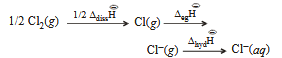The energy involved in the conversion of    (using data ,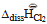= 240 kJ mol-1  ,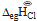= = -349 kJ mol-1,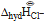= -381 kJ mol-1)   will be

• Option 1)

+120 kJ mol-1

• Option 2)

+152 kJ mol-1

• Option 3)

-610 kJ mol-1

• Option 4)

-850 kJ mol-1

As we learnt in Bond dissociation enthalpy - It is the average of enthalpy required to dissociate the said bond present in different gaseous compound in to free atoms in gaseous state. - wherein     Enthalpy of Hydration - Amount of enthalpy evolved during hydration of 1 mole anhydrous compound. - wherein    The energy involved in the conversion of to is given Substituting various values...
Engineering
99 Views   |

Standard entropy of   are 60, 40 and 50 J K-1 mol-1, respectively. For the reaction,

to be at equilibrium, the temperature will be

• Option 1)

1000 K

• Option 2)

1250 K

• Option 3)

500 K

• Option 4)

750 K

As we learnt in  Gibb's free energy (Δ G) -   - wherein Gibb's free energy enthalpy of reaction entropy temperature     Entropy for isothermal process - or   - wherein     For a reaction to be equilibrium  since  so at equilibrium  For reaction Calculating  for the above equation, we get  At equilibrium,  Option 1) 1000 K This option is incorrect  Option 2) 1250 K This option...
Engineering
117 Views   |

Let . Then which one of the following is true?

• Option 1)

• Option 2)

• Option 3)

• Option 4)

As we learnt in Properties of Definite Integration - If for all then -     So,  Now,    Option 1) Incorrect Option 2) Incorrect Option 3) Correct Option 4) Incorrect
Engineering
1145 Views   |

An experiment is performed to find the refractive index of glass using a travelling microscope. In this experiment distances are measured by

• Option 1)

a screw gauge provided on the microscope

• Option 2)

a vernier scale provided on the microscope

• Option 3)

a standard laboratory scale

• Option 4)

a meter scale provided on the microscope.

As we learnt in. To determine refractive index of a glass slab using travelling microscope - Calculation Result:  It gives R.I of material of the glass slab. * Const. of proportionality in Snell's Law is called R.I.        - wherein < SPQ = i sini =  <SP1 Q=r  sinr =  Procedure  1.    The least of the vertical scale of the travelling microscope are determined. 2.    Make a black-ink...
Engineering
526 Views   |

Two full turns of the circular scale of a screw gauge cover a distance of 1 mm on its main scale. The total number of divisions on the circular scale is 50. Further, it is found that the screw gauge has a zero error of – 0.03 mm. While measuring the diameter of a thin wire, a student notes the main scale reading of 3 mm and the number of circular scale divisions in line with the main scale as 35. The diameter of the wire is

• Option 1)

3.38 mm

• Option 2)

3.32 mm

• Option 3)

3.73 mm

• Option 4)

3.67 mm.

As we learnt in To measure the diameter of small spherical cylindrical body using Vernier Callipers - Vernier Constant = 1 Main scale division - 1 V.S. Division  V.C= 1 M.S.D - 1 V.S.D M.S.D=  Main Scale Reading V.S.D= Vernier Scale Reading   - wherein Total observed reading =  N= Nth division  Observations:  1.    Vernier constant (least count) of the Vernier Callipers:                     ...
Engineering
114 Views   |

A horizontal overhead powerline is at a height of 4 m from the ground and carries a current of 100 A from east to west. The magnetic field directly below it on the ground is

• Option 1)

• Option 2)

• Option 3)

• Option 4)

As we learnt in Ampere's Law -   Total Current crossing the above area is  - wherein Outward postive    Inward negative X     from Ampers's theorem Option 1) Incorrect Option 2) Incorrect Option 3) Incorrect Option 4) Correct
Engineering
107 Views   |

Relative permittivity and permeability of a material are ,  respectively. Which of the following values of these quantities are allowed for a diamagnetic mateiral?

• Option 1)

• Option 2)

• Option 3)

• Option 4)

As we learnt in Relative Permeability - - wherein  - relative permeability of any medium  - relative permeability of air or vaccum     Magnetic Permeability - - wherein  - absolute permeability of air  - relative permeability tesla m/amp     The values of relative permeability of diamagnetic materials are slightly less than 1 and is quite high. According to the table given, one takes ...
Engineering
1026 Views   |

A parallel plate capacitor with air between the plates has a capacitance of  9pF. The separation between its plates is d . The space between the plates is now filled with two dielectrics. One of the dielectrics has dielectric constan  k1 = 3  and thickness  d/3 while the other one has dielectric constant k2 = 6 and thickness 2d/3   Capacitance of the capacitor is now

• Option 1)• Option 2)• Option 3)• Option 4)As we learnt in

Parallel Plate Capacitor -

- wherein

Area - A seperation between two plates.

If K filled between the plates -

- wherein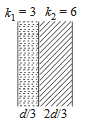With dielectric,

(as they are in series)

Option 1)Incorrect

Option 2)Incorrect

Option 3)Incorrect

Option 4)Correct

View More
Exams
Articles
Questions